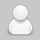# 高效使用 Python 可视化工具 Matplotlib

www.ft12.com6年前 (2017-07-04)短网址资讯1373

Matplotlib是Python中最常用的可视化工具之一,可以非常方便地创建海量类型的2D图表和一些基本的3D图表。本文主要介绍了在学习Matplotlib时面临的一些挑战，为什么要使用Matplotlib，并推荐了一个学习使用Matplotlib的步骤。

matplotlib的新用户应该学习使用面向对象的接口。

matplotlib的另一个历史性挑战是，一些默认风格选项相当没有吸引力。 在R语言世界里，可以用ggplot生成一些相当酷的绘图，相比之下，matplotlib的选项看起来有点丑。令人欣慰的是matplotlib 2.0具有更美观的样式，以及非常便捷对可视化的内容进行主题化的能力。

1. 学习基本的matplotlib术语，尤其是什么是图和坐标轴

2. 始终使用面向对象的接口，从一开始就养成使用它的习惯

3. 用基础的pandas绘图开始你的可视化学习

4. 用seaborn进行更复杂的统计可视化

5. 用matplotlib来定制pandas或者seaborn可视化

import pandas as pd

import matplotlib.pyplot as plt

from matplotlib.ticker import FuncFormatter

top_10 = (df.groupby('name')['ext price', 'quantity'].agg({'ext price''sum', 'quantity''count'})

.sort_values(by='ext price', ascending=False))[:10].reset_index()

top_10.rename(columns={'name''Name', 'ext price''Sales', 'quantity''Purchases'}, inplace=True)

plt.style.available

['seaborn-dark',

'seaborn-dark-palette',

'fivethirtyeight',

'seaborn-whitegrid',

'seaborn-darkgrid',

'seaborn',

'bmh',

'classic',

'seaborn-colorblind',

'seaborn-muted',

'seaborn-white',

'seaborn-talk',

'grayscale',

'dark_background',

'seaborn-deep',

'seaborn-bright',

'ggplot',

'seaborn-paper',

'seaborn-notebook',

'seaborn-poster',

'seaborn-ticks',

'seaborn-pastel']

plt.style.use('ggplot')

top_10.plot(kind='barh', y="Sales", x="Name")

fig, ax = plt.subplots()

top_10.plot(kind='barh', y="Sales", x="Name", ax=ax)

fig, ax = plt.subplots()

top_10.plot(kind='barh', y="Sales", x="Name", ax=ax)

ax.set_xlim([-10000, 140000])

ax.set_xlabel('Total Revenue')

ax.set_ylabel('Customer');

fig, ax = plt.subplots()

top_10.plot(kind='barh', y="Sales", x="Name", ax=ax)

ax.set_xlim([-10000, 140000])

ax.set(title='2014 Revenue', xlabel='Total Revenue', ylabel='Customer')

fig, ax = plt.subplots(figsize=(5, 6))

top_10.plot(kind='barh', y="Sales", x="Name", ax=ax)

ax.set_xlim([-10000, 140000])

ax.set(title='2014 Revenue', xlabel='Total Revenue')

ax.legend().set_visible(False)

def currency(x, pos):

'The two args are the value and tick position'

if x >= 1000000:

return '\${:1.1f}M'.format(x*1e-6)

return '\${:1.0f}K'.format(x*1e-3)

fig, ax = plt.subplots()

top_10.plot(kind='barh', y="Sales", x="Name", ax=ax)

ax.set_xlim([-10000, 140000])

ax.set(title='2014 Revenue', xlabel='Total Revenue', ylabel='Customer')

formatter = FuncFormatter(currency)

ax.xaxis.set_major_formatter(formatter)

ax.legend().set_visible(False)

# Create the figure and the axes

fig, ax = plt.subplots()

# Plot the data and get the averaged

top_10.plot(kind='barh', y="Sales", x="Name", ax=ax)

avg = top_10['Sales'].mean()

# Set limits and labels

ax.set_xlim([-10000, 140000])

ax.set(title='2014 Revenue', xlabel='Total Revenue', ylabel='Customer')

# Add a line for the average

ax.axvline(x=avg, color='b', label='Average', linestyle='--', linewidth=1)

# Annotate the new customers

for cust in [3, 5, 8]:

ax.text(115000, cust, "New Customer")

# Format the currency

formatter = FuncFormatter(currency)

ax.xaxis.set_major_formatter(formatter)

# Hide the legend

ax.legend().set_visible(False)

fig, (ax0, ax1) = plt.subplots(nrows=1, ncols=2, sharey=True, figsize=(7, 4))

# Get the figure and the axes

fig, (ax0, ax1) = plt.subplots(nrows=1,ncols=2, sharey=True, figsize=(7, 4))

top_10.plot(kind='barh', y="Sales", x="Name", ax=ax0)

ax0.set_xlim([-10000, 140000])

ax0.set(title='Revenue', xlabel='Total Revenue', ylabel='Customers')

# Plot the average as a vertical line

avg = top_10['Sales'].mean()

ax0.axvline(x=avg, color='b', label='Average', linestyle='--', linewidth=1)

# Repeat for the unit plot

top_10.plot(kind='barh', y="Purchases", x="Name", ax=ax1)

avg = top_10['Purchases'].mean()

ax1.set(title='Units', xlabel='Total Units', ylabel='')

ax1.axvline(x=avg, color='b', label='Average', linestyle='--', linewidth=1)

# Title the figure

fig.suptitle('2014 Sales Analysis', fontsize=14, fontweight='bold');

# Hide the legends

ax1.legend().set_visible(False)

ax0.legend().set_visible(False)Matplotlib支持许多不同格式文件的保存。 你可以用fig.canvas.get_supported_filetypes（）查看系统支持的格式：

fig.canvas.get_supported_filetypes()

{'eps''Encapsulated Postscript',

'jpeg''Joint Photographic Experts Group',

'jpg''Joint Photographic Experts Group',

'pdf''Portable Document Format',

'pgf''PGF code for LaTeX',

'png''Portable Network Graphics',

'ps''Postscript',

'raw''Raw RGBA bitmap',

'rgba''Raw RGBA bitmap',

'svg''Scalable Vector Graphics',

'svgz''Scalable Vector Graphics',

'tif''Tagged Image File Format',

'tiff''Tagged Image File Format'}

fig.savefig('sales.png', transparent=False, dpi=80, bbox_inches="tight")### 屈臣氏要开始在网上卖药了 凑热闹还是玩真的？

【FT12短网址】屈臣氏在2013年推出了自有电商渠道，在该渠道上能够搞定你所有需求的平时用品，从洗护用品、彩妆、食品、保健品，再到手机配件、床上用品等包罗万象。接下来屈臣氏也许想要帮你把药品及医疗器械的疑问也处理了。有业内人士告诉亿邦动力...

### 优胜略汰，7个在 iOS 11 上被移除的功能

iOS 11 上增加的很多新功能我们都已经有所了解，不过，在新版系统当中，苹果同样也移除和淘汰了一些认为对用户体验帮助不大的功能，而且不易被用户察觉。本文我们就为大家综合了多个在 iOS 11 上被移除的功能，看看对你来说是不是都无用。1、...

#### 发表评论◎欢迎参与讨论，请在这里发表您的看法和观点。